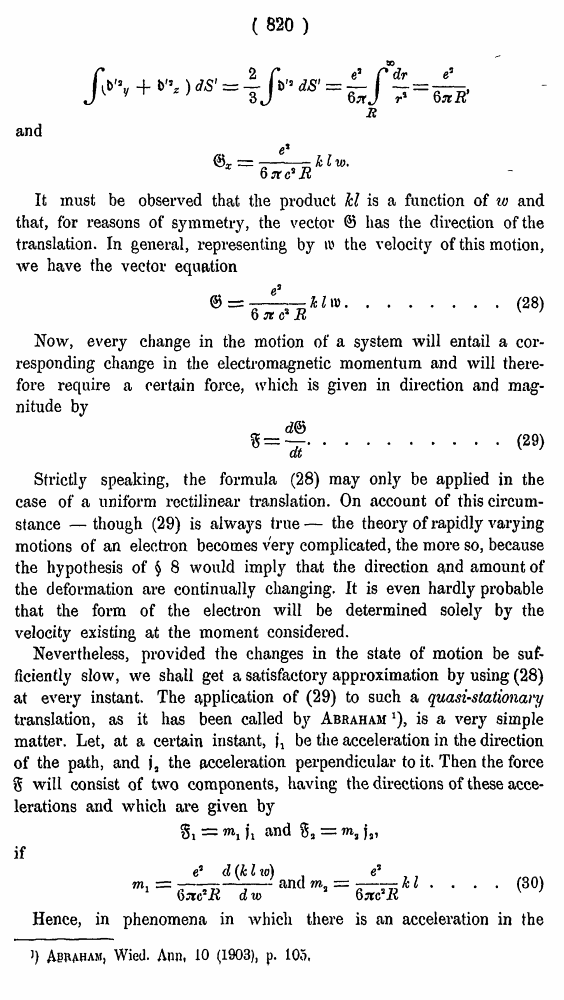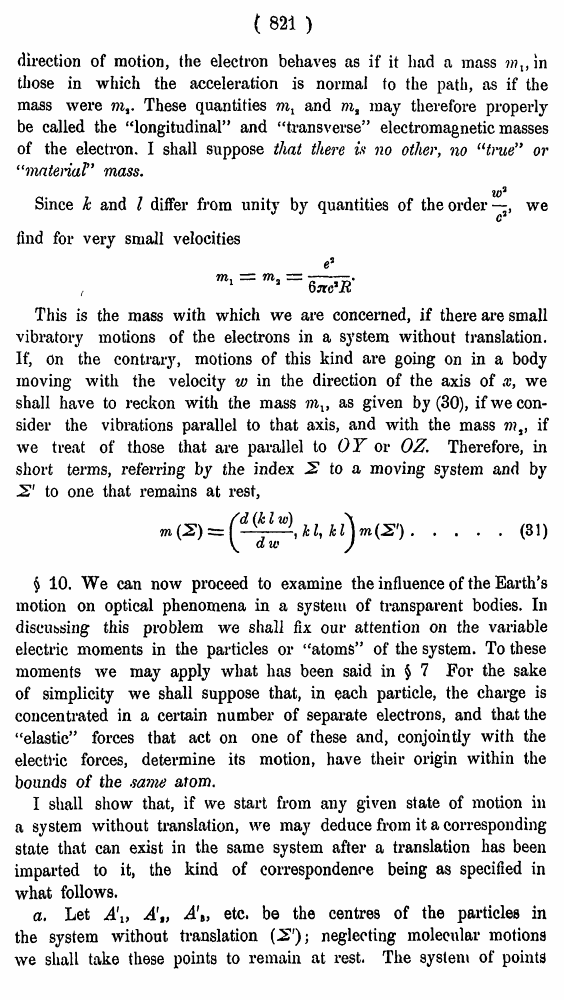# H. A. Lorentz. Electromagnetic phenomena in a system moving with any velocity smaller than that of light. // Proceedings Royal Acad., Amsterdam. Vol. VI., 1904

В начало   Другие форматы   <<<     Страница 820   >>>809  810  811  812  813  814  815  816  817  818  819  820 821  822  823  824  825  826  827  828  829  830  831 ( 820 ) and It must be observed that the product hi is a function of w and that, for reasons of symmetry, the vector <3 has the direction of the translation. In general, representing by ti' the velocity of this motion, we have the vector equation .......(28) Now, every change in the motion of a system will entail a corresponding change in the electromagnetic momentum and will there-foi’e require a certain force, which is given in direction and magnitude by ........(29) Strictly speaking, the formula (28) may only be applied in the case of a uniform rectilinear translation. On account of this circumstance — though (29) is always true — the theory of rapidly varying motions of an electron becomes very complicated, the more so, because the hypothesis of § 8 would imply that the direction and amount of the deformation are continually changing. It is even hardly probable that the form of the electron will be determined solely by the velocity existing at the moment considered. Nevertheless, provided the changes in the state of motion be sufficiently slow, we shall get a satisfactory approximation by using (28) at every instant. The application of (29) to such a quasi-stationary translation, as it has been called by Abraham *), is a very simple matter. Let, at a certain instant, |\ be the acceleration in the direction of the path, and j„ the acceleration perpendicular to it. Then the force % will consist of two components, having the directions of these accelerations and which are given by if .... (30) Hence, in phenomena in which there is an acceleration in the J) Asraham, Wied. Ann, 10 (1903), p. 105, ( 821 ) direction of motion, the electron behaves as if it had a mass ???,, in those in which the acceleration is normal to the path, as if the mass were mf. These quantities ml and m% may therefore properly be called the “longitudinal” and “transverse” electromagnetic masses of the electron. I shall suppose that there is no other, no “true” or “material” mass. Since k and I differ from unity by quantities of theordeiwe find for very small velocities ( This is the mass with which we are concerned, if there are small vibratory motions of the electrons in a system without translation. If, On the contrary, motions of this kind are going on in a body moving with the velocity w in the direction of the axis of x, we shall have to reckon with the mass as given by (30), if we consider the vibrations parallel to that axis, and with the mass mt, if we treat of those that are parallel to OY or OZ, Therefore, in short terms, referring by the index 2 to a moving system and by S' to one that remains at rest, .... (31) § 10. We can now proceed to examine the influence of the Earth’s motion on optical phenomena in a system of transparent bodies. In discussing this problem we shall fix our attention on the variable electric moments in the particles or “atoms” of the system. To these moments we may apply what has been said in \$ 7 For the sake of simplicity we shall suppose that, in each particle, the charge is concentrated in a certain number of separate electrons, and that the “elastic” forces that act on one of these and, conjointly with the electlic forces, determine its motion, have their origin within the bounds of the same atom. I shall show that, if we start from any given state of motion in a system without translation, we may deduce from it a corresponding state that can exist in the same system after a translation has been imparted to it, the kind of correspondence being as specified in what follows. a. Let Ai, A'„ A\, etc. be the centres of the particles in the system without translation (2'); neglecting molecular motions we shall take these points to remain at rest. The system of points